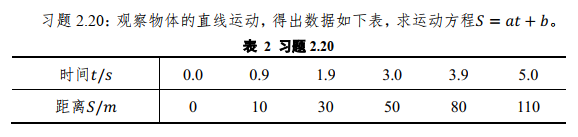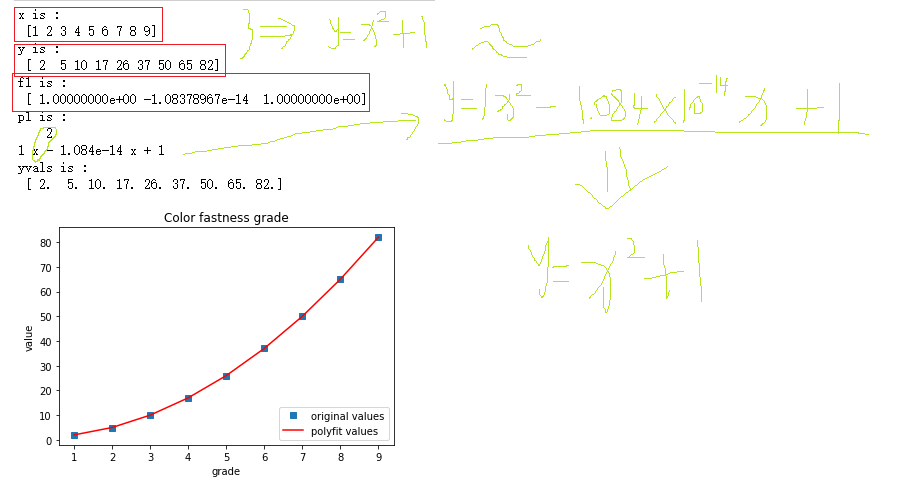• python拟合离散数据
2020-12-04 15:08:15

我是scipy和matplotlib的新手,我一直在尝试将函数与数据相匹配. Scipy Cookbook中的第一个例子非常有效,但是当我尝试从文件中读取点时,我给出的初始系数(下面的p0)似乎从未真正改变,协方差矩阵总是INF.

我试图在一条线后拟合数据,但无济于事.我导入数据的方式有问题吗？如果是这样,有没有更好的方法呢？

import matplotlib.pyplot as plt

from scipy.optimize import curve_fit

import scipy as sy

with open('data.dat') as f:

dtipus = [('x', sy.float32)] + [('y', sy.float32)]

x = data['x']

y = data['y']

def func(x, a, b, c):

return a*x**b + c

p0 = sy.array([1,1,1])

coeffs, matcov = curve_fit(func, x, y, p0)

yaj = func(x, coeffs, coeffs, coeffs)

print(coeffs)

print(matcov)

plt.plot(x,y,'x',x,yaj,'r-')

plt.show()

谢谢！

解决方法:

在我看来,问题确实在于如何导入数据.伪造这个数据文件：

$:~/temp$cat data.dat

1.0 2.0

2.0 4.2

3.0 8.4

4.0 16.1

import matplotlib.pyplot as plt

from scipy.optimize import curve_fit

import scipy as sy

import pylab as plb

x = data[:,0]

y= data[:,1]

def func(x, a, b, c):

return a*x**b + c

p0 = sy.array([1,1,1])

coeffs, matcov = curve_fit(func, x, y, p0)

yaj = func(x, coeffs, coeffs, coeffs)

print(coeffs)

print(matcov)

plt.plot(x,y,'x',x,yaj,'r-')

plt.show()

适合我.顺便说一句,您可以使用dtypes来命名列.

标签：least-squares,python,scipy,python-3-x,curve-fitting

更多相关内容
• 有一组4096长度的数据，需要找到一阶导数从正到负的点，和三阶导数从负到正的点，截取了一小段。 394.0 388.0 389.0 388.0 388.0 392.0 393.0 395.0 395.0 394.0 394.0 390.0 392.0 按照之前所了解的，...
• 多项式拟合 import numpy as np import matplotlib.pyplot as plt def cost_function(w, b, data): total_cost = 0 M = len(data) for i in range(M): x = data[i, 0] y = data[i, 1] total_cost = 0.5/M ...

## 一. 多项式拟合import numpy as np
import matplotlib.pyplot as plt

def cost_function(w, b, data):
total_cost = 0
M = len(data)
for i in range(M):
x = data[i, 0]
y = data[i, 1]
total_cost = 0.5/M * (np.square(y-w*x-b)).sum()

b_sum = 0.0
w_sum = 0.0
M = float(len(data))

# 对每个点代入公式求和
for i in range(0, len(data)):
x = data[i, 0]
y = data[i, 1]
tmp = w_current * x + b_current - y
b_sum += tmp
w_sum += tmp * x

# 用公式求当前梯度
grad_w = 2 / M * w_sum
grad_b = 2 / M * b_sum

# 梯度下降，更新当前的w和b
b_new = b_current - alpha * grad_b
w_new = w_current - alpha * grad_w
return [b_new, w_new]

def gradient_descent(data, b_start, w_start, alpha, num_iterations):
b = b_start
w = w_start
# 保存所有的损失函数值，用来显示下降过程
cost_list=[]
for i in range(num_iterations):
cost_list.append(cost_function(w, b, data))
b_new, w_new = step_gradient(b, w, data, alpha)
b = b_new
w = w_new
return [b, w, cost_list]

#  create data
b_start = 1
w_start = 1
alpha = float(input("步长："))
num_iterations = int(input("迭代次数："))
data = [
[0.0, 0],
[0.9, 10],
[1.9, 30],
[3.0, 50],
[3.9, 80],
[5.0, 110]
]
data = np.array(data)
x = data[:, 0]
y = data[:, 1]

# train
b_now, w_now, cost_list = gradient_descent(data, b_start, w_start, alpha, num_iterations)
print("w is :", w_now)
print("b is :", b_now)
print("运动方程S=%f*t+(%f)"%(w_now, b_now))

# 精确值
p = np.polyfit(x, y, deg=1)
print("参考系数:", p)

# plot
plt.scatter(x, y)
ori_y = x * w_start + b_start
plt.plot(x, ori_y, color="green", label="origin")
pre_y = w_now * x + b_now
plt.plot(x, pre_y, color="red", label="fit")
plt.xlabel("x")
plt.ylabel("y")
plt.legend()
plt.figure()
cost = cost_function(w_now, b_now, data)
# print("cost_list:", cost_list)
print("cost is:", cost)
plt.plot(cost_list)

plt.show()



## 二. 曲线拟合

import numpy as np
import matplotlib.pyplot as plt
from scipy.optimize import curve_fit

# 原始散点坐标
x = [70,100,120,150,180, 200]
y = [0.95,0.85,0.5,0.2,0.05, 0]

xd = np.array([70,100,120,150,180])
yd = np.log(np.array([0.95,0.85,0.5,0.2,0.05]))

# 表达式
def func(x, d, n):
return - np.power(x / d, n)

# 非线性最小二乘拟合
popt, pcov = curve_fit(func, xd, yd)

# 获取popt里的拟合系数
d = popt
n = popt

# 拟合yd的值

yvals = np.exp(func(x, d, n))
print("d:", d)
print("n:", n)

# 绘图
plt.figure()
plt.plot(x, y, 'd', label="Original Values")
xx = np.linspace(70,200,50)
y_vals = np.exp(func(xx, d, n))
plt.plot(xx, y_vals, 'r', label="$fit: y_d = e^{-(\\frac{d}{%5.5f})^{%5.5f}}$" % tuple(popt))
plt.xlabel("diameter")
plt.ylabel("mass fraction")
plt.legend(loc=1) # 图例位置
plt.show()


展开全文• 代码中，f1 = np.polyfit(x, y, 2)，2表示使用二次函数进行拟合 import numpy as np import matplotlib.pyplot as plt #定义x、y散点坐标 x = [1,2,3,4,5,6,7,8,9] x = np.array(x) print('x is :\n',x)

### 自定义离散点进行指定多项式函数拟合

##### 用户自己自己输入坐标点，拟合函数可根据用户输入的多项式的最高次方进行自动拟合函数，拟合方法采用最小二乘法进行函数拟合。

(1,2),(2,5),(3,10),(4,17),(5,26),(6,37)(7,50),(8,65),(9,82)
很显然是函数为二次函数，y=x^2+1
代码中，f1 = np.polyfit(x, y, 2)，2表示使用二次函数进行拟合

import numpy as np
import matplotlib.pyplot as plt

#定义x、y散点坐标
x = [1,2,3,4,5,6,7,8,9]
x = np.array(x)
print('x is :\n',x)

num = [2,5,10,17,26,37,50,65,82]

y = np.array(num)
print('y is :\n',y)

#用多次多项式拟合
f1 = np.polyfit(x, y, 2)
print('f1 is :\n',f1)
p1 = np.poly1d(f1)
print('p1 is :\n',p1)

#也可使用yvals=np.polyval(f1, x)
yvals = p1(x) #拟合y值
print('yvals is :\n',yvals)

#绘图
plot1 = plt.plot(x, y, 's',label='original values')
plot2 = plt.plot(x, yvals, 'r',label='polyfit values')
plt.ylabel('value')
plt.legend(loc=4) #指定标识的位置(1为右上角，2为左上角，3为左下角，4为右下角)
plt.show()


运行效果如下：展开全文• 前言：本文详细介绍如何在Python拟合Logit模型，包括数据准备、哑变量的处理、参数拟合结果解读等内容。本文为系列离散选择模型(DiscreteChoice Model, DCM)系列文章的第6篇。更多文章请关注公众号：蜂蜂Eric。在...

前言：本文详细介绍如何在Python中拟合Logit模型，包括数据准备、哑变量的处理、参数拟合结果解读等内容。

本文为系列离散选择模型(Discrete

Choice Model, DCM)系列文章的第6篇。更多文章请关注公众号：蜂蜂Eric。

在掌握Logit模型的基本理论框架之后，可以通过多种方法进行模型的拟合——SAS、R、MATLAB、Stata、Python都可以。在DCM系列文章的第5篇中，我们用SAS软件去拟合Logit模型(更多介绍请参照：Logit模型拟合实战案例(SAS)——离散选择模型之五)；本篇尝试用Python去拟合同样的模型，并对结果进行解读。案例介绍：

这里仍然使用和上一篇中相同的数据。我们要研究的问题是：在申请的研究生的时候，什么样的学生更容易被录取。

原始数据保存在名为“Application.csv”的文件中(文件格式为csv格式)，每一行代表一条申请者的记录：原始数据中包含3个自变量：

申请者的GRE成绩，用变量gre表示；

申请者的平均绩点，用变量gpa表示；

申请者所在的本科院校的排名，用变量rank表示。

变量gre和gpa都是连续变量。rank为离散变量，只能取1、2、3、4中的某一个值；rank=1对应的学校排名最高，而rank=4对应的排名最低。

软件准备：

本例需要调用下面这几个包：

numpy：Python中用于数值计算的包，可以方便地进行数组和矩阵的相关计算；

pandas：利用pandas可以高效地对数据进行操作和管理；

statsmodels：Python中用于统计建模和计量经济学的包，可以进行描述性统计、统计模型估计和推断等操作；

pylab：本例中用于绘图。

运行Python代码之前，请确保已经正确安装相应的软件包。

建模准备：

正式建模之前，可以先做一些描述性分析(Descriptive analysis)——看一看样本中各变量的均值、方差等等，以加强对数据的理解。具体实现步骤如下。

在Python中导入相应的包：函数读取原始数据文件，并展示前5行：由于pandas的DataFrame数据结构也有一个方法的名称为rank，这容易与原始数据表中的列名rank产生混淆。将原始数据表中的列名rank更改为sch_rank：用describe() 函数对样本中的各变量做描述性分析，结果如下面所示。我们可以得到每一个变量的出现的频数(count)、均值(mean)、标准差(std)、最大/小值(min/max)、百分位数(25%，50%，75%)等信息。这一步相当于SAS中的Proc

Means和Proc Freq。当然，还可以做一下交叉频数分析，粗略地观察(离散的)自变量和因变量之间关系。例如，根据下图我们就可以看出：在样本中，当申请者所在的学校排名越高时(’sch_rank=1’)，申请者被录取的比例也就越大。还可以利用直方图来可视化数据：(P.S. Python新手一枚，这图中间有点挤，各位有什么方法可以增加中间的间距么？谢谢！)

数据准备：

在Python中拟合Logit模型的过程非常简单，直接调用statsmodels库中的Logit() 函数即可。调用Logit() 函数的基本格式：Logit() 函数有两个输入参数：exog代表和自变量(X)对应的数据；本例中就是变量gre、gpa、rank(后更名为sch_rank)相对应的那一部分数据：问题在于——

(1)变量sch_rank是一个分类变量，需要对其进行哑变量处理。在SAS中，分类变量的哑变量化是通过 class 语句实现的(如下图)，而在Python中这一步需要手动实现。(2)Logit()函数不会自动添加常数项，因此我们在准备数据的时候，需要手动添加常数项。可见，知道了Python中利用Logit() 函数就可以拟合Logit模型后，剩余工作的难点在于数据的准备。

利用pandas中的get_dummies()

函数对分类变量sch_rank进行哑变量化操作，其结果是得到sch_rank_1、sch_rank_2、sch_rank_3、sch_rank_4四个0-1变量：由于sch_rank_1+ sch_rank_2 + sch_rank_3 + sch_rank_4=

1, 所以不能直接把这四个变量同时放到模型(否则会有共线性的问题)，我们选取sch_rank_4作为基变量(和上一篇的SAS案例保持一致)，把sch_rank_1、sch_rank_2、sch_rank_3和其它两个自变量gre、gpa的数据拼到一起：手动添加常数项：至此，数据准备工作已经完成！

模型拟合：

在拟合Logit模型的时候，只要从上面的data中提取出因变量、自变量(含常数项)相对应的列，然后放到Logit()函数中即可。

提取和自变量、常数项相对应的列名：拟合Logit模型。拟合的结果存储于result对象中：输出result对象中的拟合结果：上表中输出了Logit模型的相关拟合结果。结果包含两部分：上半部分给出了和模型整体相关的信息，包括因变量的名称(Dep. Variable: admit)、模型名称(Model: Logit)、拟合方法(Method: MLE 最大似然估计)等信息；下半部分则给出了和每一个系数相关的信息，包括系数的估计值(coef)、标准误(std err)、z统计量的值、显著水平(P>|z|)和95%置信区间。

根据上表可以得到本例中Logit模型的具体形式：由于哑变量sch_rank_3的值并不显著(0.591)，因此sch_rank_3没有包含在上面的模型中。

前文中说过(参见系列文章之：Logit究竟是个啥？–离散选择模型之三 – 知乎专栏)，在Logit模型中，变量的系数是指：自变量每变化一个单位，胜率(Odds)的对数的变化值。在本例中，以变量gre的系数为例，其解读方式为：

当其它变量保持不变时，申请者的GRE成绩每增加一分，其被录取的胜率的对数增加0.0023。取对数后，可以得到胜率变成原来的倍(这一步的计算代码参见下图)。

求各系数的指数值(即相应的Odds)：也可输出和Odds相对应的95%置信区间：我们可以将Python中输出的结果和SAS中的结果(见下图)进行对比——二者的系数估计结果基本一致(一个细小的区别是：在检验单个变量是否显著时，statsmodels用的是z统计量，SAS用的是Wald Chi-Square 统计量)。更多关于离散选择模型的文章，欢迎关注微信公众号：蜂蜂Eric。

参考资料：

更多相关文章请关注微信公众号：蜂蜂Eric

展开全文• 主要介绍了使用python实现离散时间傅里叶变换的方法，文中通过示例代码介绍的非常详细，对大家的学习或者工作具有一定的参考学习价值，需要的朋友们下面随着小编来一起学习学习吧
• 使用 scipy.signal 的 argrelextrema 函数(API)，简单方便 import numpy as np import pylab as pl import matplotlib.pyplot as plt import scipy.signal as signal x=np.array([ 0, 6, 25, 20, 15, 8, 15, 6, 0,...
• 第一种是进行多项式拟合，数学上可以证明，任意函数都可以表示为多项式形式。具体示例如下。 ###拟合年龄 import numpy as np import matplotlib.pyplot as plt #定义x、y散点坐标 x = [10,20,30,40,50,60,70,80] x...
• 假设我有两个数据列表，如下所示：x = [1, 2, 3, 4, 5, 6, 7, 8, 9, 10]y = [1, 2, 3, 4, 5, 6, 8, 10, 12, 14]也就是说，很明显，仅仅将一条直线拟合到这些数据上是行不通的，而是在数据中的某个点改变了坡度。...
• 三维空间中的多个点进行直线拟合python程序后期进行了优化，更直观显示，里面增加了halcon代码，便于放大后查看离散点对于直线位置的偏移情况。halcon 计算机视觉
• 不过，恋习python突然想到，可以通过python将故宫的建筑物图片，转化为手绘图（素描效果）。 效果图如下：? 一、概念与原理我们都知道手绘图效果的特征...根据灰度变化来模拟人类视觉的模拟程度把图像看成二维离散...
• Python曲线拟合_Jak的博客-CSDN博客_python 曲线拟合 import numpy as np import matplotlib.pyplot as plt from pylab import mpl mpl.rcParams['font.sans-serif'] = ['SimHei'] # 指定默认字体 plt.rcP..开发语言 后端
• 第一种是进行多项式拟合，数学上可以证明，任意函数都可以表示为多项式形式。具体示例如下。 ###拟合年龄 import numpy as np import matplotlib.pyplot as plt   #定义x、y散点坐标 x = [10,20,30,40,50,60,70,80...
• 今天小编就为大家分享一篇Python实现二维曲线拟合的方法，具有很好的参考价值，希望对大家有所帮助。一起跟随小编过来看看吧
• 直方图是用于展示数据的分组分布状态的一种图形，用矩形的宽度和高度表示频数分布，通过直方图，用户可以很直观的看出数据分布的形状、中心位置以及数据离散程度等。 在python中一般采用matplotlib库的hist来绘制...
• I'm trying to fit a sigmoid function to some data I have but I keep getting:ValueError: Unable to determine number of fit parameters.My data looks like this:My code is:from scipy.optimize import curve...
• 这是个复杂的问题，没有完美的答案，对于给定的数据，有两种设置概率分布函数参数的方法：在我的经验中，最近几年最大似然是首选的，尽管这可能不是每个领域的情况。这是如何估算R中参数的具体示例。考虑从高斯分布...
• 然后今天有个课程设计的报告，上去讲了一下RANSAC，感觉这个东西也没那么复杂，所以今天就总结一些RASAC并用Python实现一下直线拟合。RANSAC简介RANSAC(RAndom SAmple Consensus,随机采样一致)算法是从一组含有...
• 需要对数据进行函数拟合，首先画一下二维散点图，目测一下大概的分布，所谓正态分布，就是高斯分布，正态曲线是一种特殊的高斯曲线。python的scipy.optimize包里的curve_fit函数来拟合曲线，当然还可以拟合很多类型...
• 主要给大家介绍了关于python中matplotlib实现最小二乘法拟合的相关资料，文中通过示例代码详细介绍了关于最小二乘法拟合直线和最小二乘法拟合曲线的实现过程，需要的朋友可以参考借鉴，下面来一起看看吧。
• # numpy来实现数据拟合 import matplotlib.pyplot as plt import numpy as np x = np.linspace(300,400,20) y = x + np.random.randint(5,20,20) #随机取5到10中间20个数 poly = np.polyfit(x,y,deg=1) z = np....机器学习
• 0.2,0.5,840)#横轴 yy=x_test[3,0,:]#纵轴-0.2s~0.5s的840个数据点 plt.scatter(t,yy,marker=".") plt.show() #画插值拟合的曲线图 f=interp1d(t,yy,kind="cubic")'linear','zero', 'slinear', 'quadratic', 'cubic'...
• 主要介绍了Python实现的多项式拟合功能,结合实例形式分析了Python基于matplotlib模块进行数值运算与图形绘制相关操作技巧,需要的朋友可以参考下
• 我们经常使用傅里叶变换来计算数字信号的频谱，进而分析数字信号，离散时间傅里叶变换的公式为：X(ejw)=∑n=−∞n=+∞x[n]e−jwn X ( e j w ) = ∑ n = − ∞ n = + ∞ x [ n ] e − j w n可是自己动手实现一遍才是...
• 离散数据的基础上补插连续函数，使得这条连续曲线通过全部给定的离散数据点。插值是离散函数逼近的重要方法，利用它可通过函数在有限个点处的取值状况，估算出函数在其他点处的近似值。插值:用来填充图像变换时...回归 机器学习
• 本文实例讲述了Python实现曲线拟合操作。分享给大家供大家参考，具体如下：这两天学习了用python拟合曲线。一、环境配置本人比较比较懒，所以下载的全部是exe文件来安装，安装按照顺利来安装。自动会找到python的......